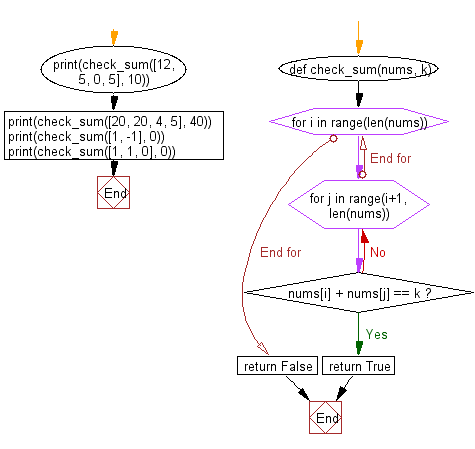﻿ Python: Check whether the sum of any two numbers from the list is equal to k or not - w3resource

# Python: Check whether the sum of any two numbers from the list is equal to k or not

## Python Basic - 1: Exercise-64 with Solution

Given a list of numbers and a number k, write a Python program to check whether the sum of any two numbers from the list is equal to k or not.
For example, given [1, 5, 11, 5] and k = 16, return true since 11 + 5 is 16.

Sample Solution:

Python Code:

``````def check_sum(nums, k):
for i in range(len(nums)):
for j in range(i+1, len(nums)):
if nums[i] + nums[j] == k:
return True
return False
print(check_sum([12, 5, 0, 5], 10))
print(check_sum([20, 20, 4, 5], 40))
print(check_sum([1, -1], 0))
print(check_sum([1, 1, 0], 0))
``````

Sample Output:

```True
True
True
False
```

Pictorial Presentation:Flowchart:Python Code Editor:

Have another way to solve this solution? Contribute your code (and comments) through Disqus.

What is the difficulty level of this exercise?

Test your Programming skills with w3resource's quiz.

﻿